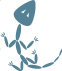libconfig.m4 at [5e72879d0d]

## File aclocal/libconfig.m4 artifact d46c22e181 part of check-in 5e72879d0d

``````AC_DEFUN(DC_DO_WIN32, [
])

AC_DEFUN(DC_DO_TYPE, [
if test -z "\$ac_cv_sizeof_long"; then
AC_C_INLINE
AC_CHECK_SIZEOF(long long, 8)
AC_CHECK_SIZEOF(long, 4)
AC_CHECK_SIZEOF(int, 4)
AC_CHECK_SIZEOF(short, 2)
fi
FOUND=0
for dc_cv_loop in \\$ac_cv_sizeof_long_long \\$ac_cv_sizeof_int \\$ac_cv_sizeof_long \\$ac_cv_sizeof_short; do
dc_cv_size=`eval echo \$dc_cv_loop`
dc_cv_name=`echo \$dc_cv_loop | sed s/\\\\$ac_cv_sizeof_//`
if test "\$dc_cv_size" = "\$3"; then
if test "\$dc_cv_name" = "int"; then
AC_CHECK_TYPE(\$1, \$2 int)
fi
if test "\$dc_cv_name" = "long"; then
AC_CHECK_TYPE(\$1, \$2 long)
fi
if test "\$dc_cv_name" = "long_long"; then
AC_CHECK_TYPE(\$1, \$2 long long)
fi
if test "\$dc_cv_name" = "short"; then
AC_CHECK_TYPE(\$1, \$2 short)
fi
FOUND=1
break
fi
done
])

SMALL=0
AC_ARG_ENABLE(small, AC_HELP_STRING([--enable-small], [Enable small build of libconfig. (disabled)]), [
case \$enableval in
yes)
SMALL=1
;;
esac
])

if test \$SMALL = 0; then
dnl Use opennet if it's available AND not small
DC_ASK_OPTLIB(opennet, fopen_net, opennet.h, [      Enable opennet support (auto)], libopennet, HAVE_LIBOPENNET, HAVE_OPENNET_H)
else
AC_DEFINE(ENABLE_SMALL, , [Define to 1 if you want to produce a minimalistic build.])
fi
])
```
```